# IAS Exam:IAS Prelims CSAT General Mental Ability Set 1

For the aspirants of IAS Prelims Exam 2016, here we have provided the questions of General Mental Ability for the Civil Services CSAT Paper II of UPSC IAS Exam 2016. The pattern of these General Mental Ability Multiple Choice Questions has been kept in line with the questions asked in earlier attempts of Civil Services IAS Exam.In the Civil Services Prelim Examination, the CSAT paper has been made qualifying in nature from 2015 onwards. The candidates have to score at least qualifying marks in the paper but in case, if a candidate could not be able to score the required qualifying marks in CSAT Paper then his/her other papers will not be evaluated. So, it is important for the candidates to do preparation in such a manner that there must not lay them in any suspicion of underscoring.

Here, we have provided the questions of General Mental Ability for the Civil Services CSAT Paper II of UPSC IAS Exam 2016. The pattern of these General Mental Ability Multiple Choice Questions has been kept in line with the questions asked in earlier attempts of Civil Services IAS Exam.

3rd set of General mental ability for CSAT Exam 2016 (20 questions) – 21st March 2016

Category: Puzzle

1. In a birthday party, each child eats at most 5 toffees. No child eats less than three biscuits. Considering all children, there are more toffees than biscuits, more biscuits than chocolates and more chocolates than number of children. Find the minimum number of children present at the party?

(a) 2
(b) 3
(c) 5
(d) 7

Explanation:

Let p, q, r and s be the number of toffees, biscuits, chocolates and children respectively.
According to question, p > q > r > s
Minimum number of children can be 2 i.e. s > 2, accordingly, p≤4
But according to question, p≥5, therefore s cannot be taken as 2.
Considering s = 3, accordingly p≤6, which can satisfy p≥5
Thus, minimum number children at party will be 3.

Category: Puzzle

2. In a cinema hall, 8 friends, H, I, J, K, L, M, N, O are sitting in a straight line according to the following rules:

• J is the neighbour of K.
• H is just next to the left of L.
• I is in one of the two seats in the middle.
• M is the only one sitting between I and L.

If N is not sitting at the extreme end, then who occupies the extreme right seat?

(a) H
(b) J
(c) K
(d) None of these

Explanation:

The possible solutions are:       N/O     M      O/N    J/K     K/J
Thus, Either J or K occupies extreme right seat.

Category: Puzzle

3. Which does not belong in this sequence?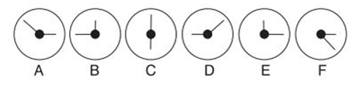(a) C
(b) B
(c) F
(d) E

Explanation:

In the others the long hand moves 45° clockwise and the short hand moves 90° clockwise.

Category: Figure matrix

4. Consider the following matrix: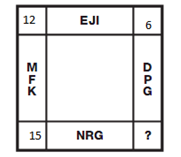What is the missing number in the given matrix?

(a) 15
(b) 18
(c) 21
(d) 19

Explanation:

The value at each corner of the diagram equals the sums of difference of the numerical values of the letters in the boxes adjacent to the corner in clockwise direction starting from NRG.

Category: Series

5. Which number replaces the question mark ?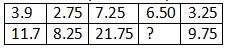(a) 11.5
(b) 12.25
(c) 13.5
(d) 9.5

Explanation: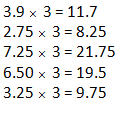Category: Puzzle

6.Find the number of triangles in the given figure?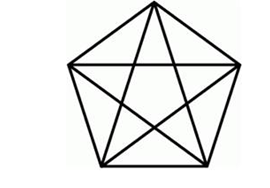(a) 32
(b) 36
(c) 35
(d) None of these

7. How many colours are required at the minimum to paint the given figure such that no two adjacent regions have the same colour? (Regions sharing a vertex should not be considered as adjacent regions)?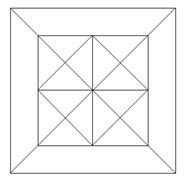(a) 2
(b) 3
(c) 4
(d) 5

Explanation: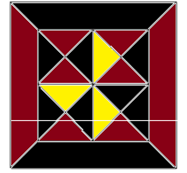Category: Puzzle

8. A dice is given in (X), when it is folded which of I, II, II and IV will be a possible figure?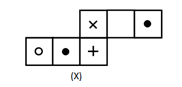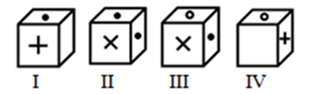(a) I, II and III
(b) I and III
(c) II and IV
(d) I, II and IV

9.In the following question, select a figure from amongst four alternatives, which figure placed in blank space of figure X would complete the pattern.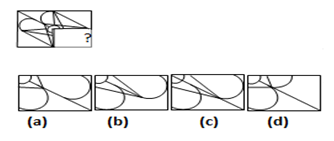10. What will come in place of question mark?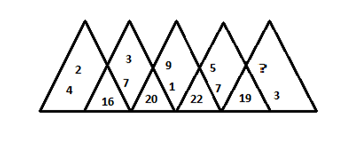(a) 4
(b) 7
(c) 5
(d) 3

Explanation:

Each number in the segment at the bottom is the sum of the four numbers in the sections either side.
Thus 5 + 7 +? + 3 = 19.
? = 4